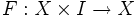# Sudden homotopy

A sudden homotopy on a topological space$X$ is a continuous map$F:X \times I \to X$ such that$F(x,0) = x \ \forall \ x$ and for any$t < 1$ the map$x \mapsto F(x,t)$ is a homeomorphism from$X$ to$X$.